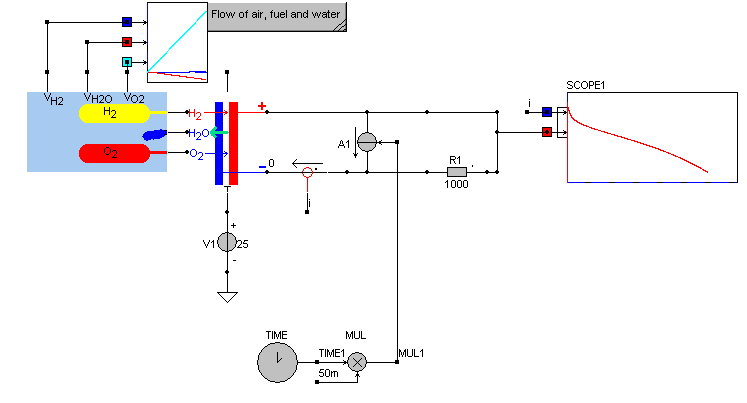• Introduction
• What is in this manual
• What is Caspoc
• User interface
• Introduction
• Starting
• Simulation
• Editing
• Viewing and printing
• Getting Started
• Basic editing
• Simulation in the time domain
• Basic User Interface Topics
• Editing
• Simulation
• Viewing
• Library
• Reports
• Project management
• Circuit and Block Diagram Components
• Introduction
• Cscript and user defined functions
• Component parameters
• Modeling Topics
• Introduction
• Power Electronics
• Semiconductors
• Electrical Machines
• Electrical drives
• Power Systems
• Mechanical Systems
• Thermal Systems
• Magnetic Circuits
• Green Energy
• Coupling to FEM
• Experimenter
• Analog hardware description language
• Embedded C code Export
• Coupling to Spice
• Small Signal Analysis
• Matlab coupling
• Tips and tricks
• Appendices

## Fuel Cell PEMC

Modeling a PEMC Fuel Cell.The model is based on the Voltage/Current Density characteristic provided by the manufacturer of the Fuel Cell.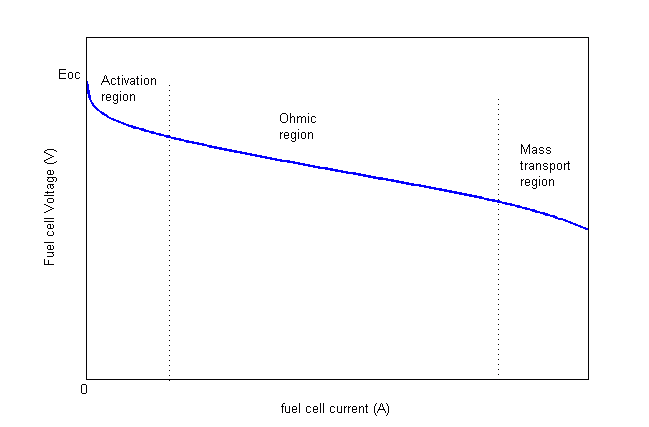The manufacturer provides this data per stack. This data is used in Caspoc and forms the basis of the Fuel Cell model.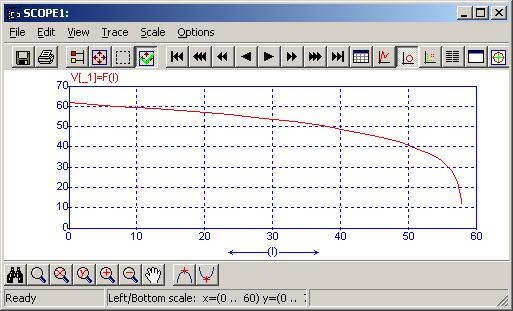On the X-axis the current density in A/cm2. The y-axis is the voltage per stack. By defining the number N of stacks and the area of the stack, the total power produced by the fuel cell can be calculated. The voltage of the fuel cell is depending on the current drawn form the fuel cell as indicated by the graph above.

The Fuel Cell library block is selected from the library at Components/Library/GreenEnergy/FuelCell

The Fuel Cell block has nine parameters, like the electrical parmeters as well as geometric parameters like the active area per cell and the number of stacks.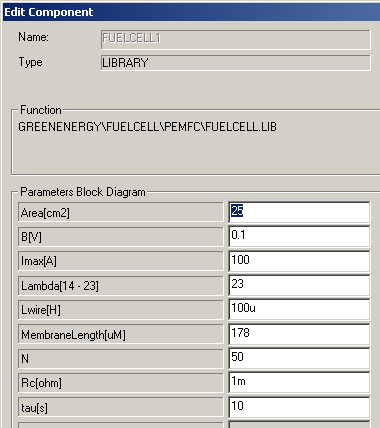The active area is given in cm2 and the number of stacks is given by the parameter N.

• Area
• The active area is smaller than the total size of the fuel cell. The current drawn from the fuel cell equals the product of the current density and the active area.
• B
• Logarithmic current voltage decay factor in the Mass Transport Region
• Imax
• Maximum current that can be withdrawn from the fuel cell
• Lambda
• Factor defining the cel membrane resistance
• Lwire
• Fuel cell internal wiring and connection inductance
• Membrane lenght
• Length of the membrane, the electrons have to cross in micro meter
• N
• The number of stacks.
• Rc
• The internal constant resistance of the fuel cell.
• tau
• Delay of the response of the fuel cell in seconds.
The influence of the time delay is clearly visible in the simulation of the load step below: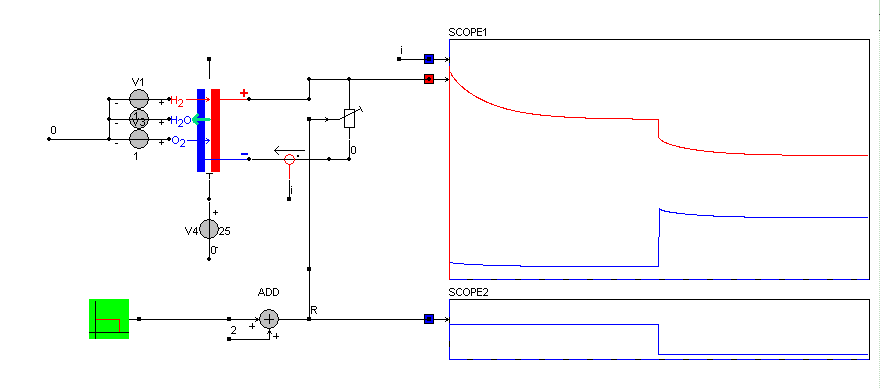Compared to the level 0 model, here the fuel and air pressure cn be defined as well as the temperature of the fuel cell. The pressure of fuel, air and water is defined by voltage sources connected to the left side of the fuel cell model. Be sure to connect the other sides of the voltage sources to a common node like ground.

The temperature is modeled by a voltage source that is connected to the bottom terminal of the block. Specify here the temperature of the fuel cell

The connections on the right side of the fuel cell are the electrical terminals. Be sure to always have nan electrical load connected as well as the temperature and air, fuel and water voltage sources.

To simplify the model a block called fuel can be used to connect to the left side of the fuel cell.